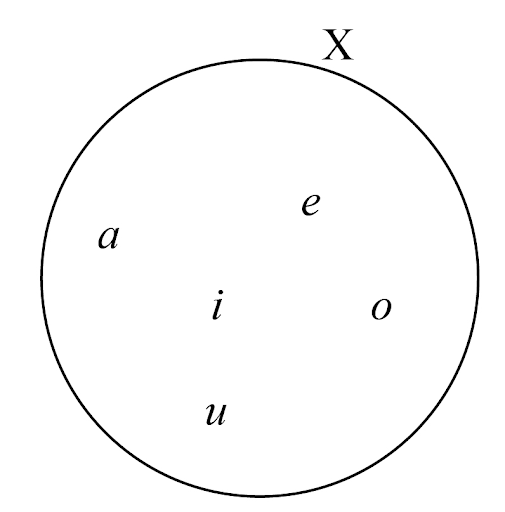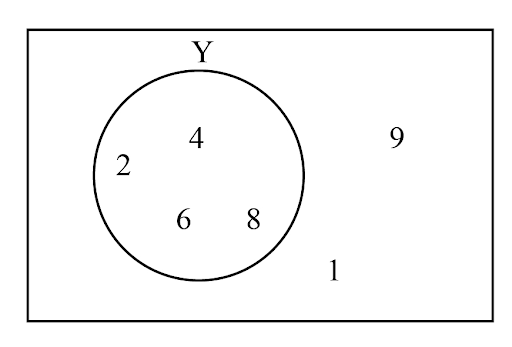# Set notation and Venn diagrams 1

Last updated: September 24, 2021

## What is set notation?

Set notation is known as a collection of elements specified to determine whether a given element is contained in a set or not. This means that $$a$$ is an element of $$X$$ or $$a$$ belongs to set $$X$$. This can be written as $$a\in X$$. The notation is $$a\notin X$$, which means that $$a$$ does not belong to $$X$$.

Set notations are the symbolic representations of elements that talk about the properties of sets.

## E1.2: Use language, notation and Venn diagrams to describe sets and represent relationships between sets

Set notation defines the elements of a set and illustrates the relationship and operations among sets. Now, let us discuss the symbols and their meanings. Let $$\in$$ mean ‘is an element of set’, $$\notin$$ mean ‘is not an element of set’, $$\left \{ \right \}$$ denote a set $$\mid$$ and $$:$$ symbols mean ‘such that’ or ‘for which’.

Now, discuss how to read and write the set notation.

### A set and its elements

A set is denoted by capital letters and the elements are represented by lowercase letters. The elements are separated by using a comma.

For example, let a set be $$X=\left \{ a, e, i, o, u \right \}$$. It can be read as a set $$X$$ that contains the English vowels.### Set membership

Set membership states if the elements are contained in a set or not. The symbol $$\in$$ is used for representing that an element is contained in a set and the symbol $$\notin$$ is used for representing that an element is not contained in a set.

For example: Let a set be $$Y=\left \{2,4,6,8 \right \}$$. So, we can write that $$2\in Y$$ means $$2$$ is an element of $$Y$$ and $$1\notinY$$ means $$1$$ is not an element of $$Y$$.### Specify member of a set

The set can be described in the set builder form as shown below:

$$P=\left \{ x\mid x\in \mathbb{R} \;\text{and}\; x\leq 3 \right \}$$ or $$P=\left \{ x: x\in \mathbb{R} \;\text{and}\; x\leq 3 \right \}$$.

It can be read that the set $$P$$ contains all the values which belong to real number and also are less than equal to $$3$$.

### Worked examples of sets and Venn diagrams

Example 1: $$X=\left \{ 1,3,5,7 \right \}$$ and $$Y=\left \{ 21,47 \right \}$$. Find $$X\cap Y$$ and $$n\left (X\cap Y \right )$$.

Step 1: Write the given values.

$$X=\left \{ 1,3,5,7 \right \}$$ and $$Y=\left \{ 21,47 \right \}$$.

Step 2: Find $$X\cap Y$$.

Since there are no common elements in both sets, the result is a null set or empty set.

So, $$X\cap Y=\left \{ \right \}$$.

Step 3: Find the value of $$n\left (X\cap Y \right )$$.

Since it is a null set, the value of $$n\left (X\cap Y \right )$$ is $$0$$.

Example 2: $$A=\left \{ 1, 2, 3, 4 \right \}$$ and $$B=\left \{ 4,5,6 \right \}$$. Find $$A\cup B$$ and also $$n\left ( A \right )$$, $$n\left ( B \right )$$ and $$n\left ( A\cup B \right )$$.

Step 1: Write the given values.

$$A=\left \{ 1, 2, 3, 4 \right \}$$ and $$B=\left \{ 4,5,6 \right \}$$.

Step 2: Find $$A\cup B$$.

$$A\cup B=\left \{ 1, 2, 3, 4, 5, 6 \right \}$$.

Step 3: Find $$n\left ( A \right )$$.

The number of elements in $$A$$ is $$4$$. Therefore, $$n\left ( A \right)=4$$.

Step 4: Find $$n\left ( B \right )$$.

The number of elements of $$B$$ is $$3$$. Therefore, $$n\left ( B \right )=3$$.

Step 5: Find $$n\left ( A\cup B \right )$$.

$$A\cup B=\left \{ 1, 2, 3, 4, 5, 6 \right \}$$.

The number of elements in $$A\cup B$$ is $$6$$. Therefore, $$n\left ( A\cup B \right )=6$$.